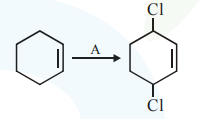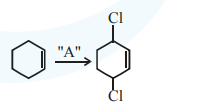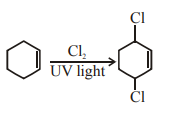# Solve this

Question:Identify the reagent(s) 'A' and condition(s) for the reaction :

1. $\mathrm{A}=\mathrm{HCl} ;$ Anhydrous $\mathrm{AlCl}_{3}$

2. $\mathrm{A}=\mathrm{HCl}, \mathrm{ZnCl}_{2}$

3. $\mathrm{A}=\mathrm{Cl}_{2} ; \mathrm{UV}$ light

4. $\mathrm{A}=\mathrm{Cl}_{2} ;$ dark, Anhydrous $\mathrm{AlCl}_{3}$

Correct Option: , 3

Solution:For substitution at allylic position in the given compound, the reagent used is $\mathrm{Cl}_{2} / \mathrm{uv}$ light.

The reaction is free radical halogenation.